# Switched Reluctance Motor for Electric Vehicles

HOME / Applications / Switched Reluctance Motor for Electric Vehicles

## Switched Reluctance Motor (SRM)

The switched reluctance motor (SRM) is a type of motor doubly salient with phase coils mounted around diametrically opposite stator poles. There are no windings or permanent magnets on the rotor. The rotor is basically a piece of (laminated) steel and its shape forms salient poles. The stator has concentrated coils.
Switched reluctance motors (SRM) have a simple and robust structure, thus they are generally suitable for high-speed applications. High-speed motors have the advantage of high power density, which is an important issue of traction motors in electric vehicles (EV). Therefore, high speed SRM seems to be promising candidates for this application.

## Solidworks model of a Switched Reluctance Motor

The designed switched reluctance motor is defined as a three phase machine, which has six inner stator poles, eight outer rotor poles and a shaft, as shown in Figure 1.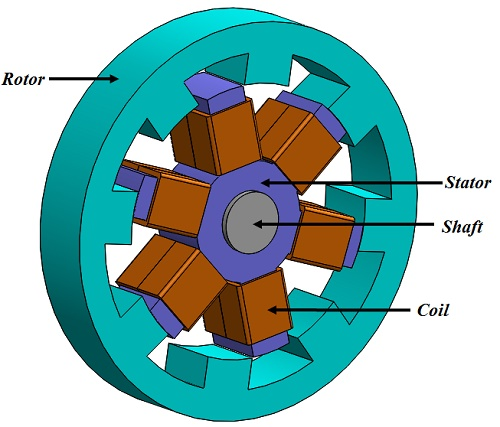Figure 1 - 3D model of a switched reluctance motor

## EMS Simulation of the In-Wheel Switched Reluctance Motor

In EMS, these types of motors are studied using Transient Magnetic simulation coupled to motion.
EMS computes the time-domain magnetic fields. The quantities for which the transient magnetic solves are the magnetic flux density, B, the magnetic field, H, and the cur­rent distribution, J; Derived quantities such as forces, torques, energy, winding loss, solid loss, flux linkage, inductance, resistance and induced voltage may be calculated from these basic field quantities.
In this simulation, Start Time, End Time and Time Increment were set to be 0 s, 0.24 s and 0.0025 s respectively.

## EMS study coupled to Solidworks

In this simulation, rotor and shaft are the moving parts. These objects are remained inside a Band, which is an Air region that encapsules completely the moving components and cannot intersect any fixed component.
The rotor and the shaft move in response to an EMF force induced by the excited coils. At each time step SolidWorks Motion will acquire the torque value from EMS and use that to determine the new position of the rotor and shaft. Then the new position is fed back to EMS and based on that the new torque value will be calculated. The cycle is then repeated for all the time steps.
To couple EMS study to Solid works, the motion analysis is selected in the Motion Manager in Solid works. Then a Torque of 0 N.m is set in the frontal face of the shaft. This torque is just a place holder and the actual value computed by EMS at each time step will be used to drive the motion of the rotor.

In EMS, a Virtual work was defined at the origin of the rotor and shaft, and coupled to the Torque defined in SolidWorks Motion study. A motion coupled EMS Study is created by coupling the Transient Magnetic study to SolidWorks Motion simulation.

This motor has 6 stator cores, excited by three phases (two stator poles comes under one phase). These Phase coils are mounted around diametrically opposite stator poles. In EMS, each phase coil (two stator windings) is modeled as a Wound coil with 120 Turns and a diameter of 9 AWG. Figure 2 illustrates the current entry Ports for the three Phase coils. Figure 3 shows the current excitation of the three coils, where the current amplitude is 45 A.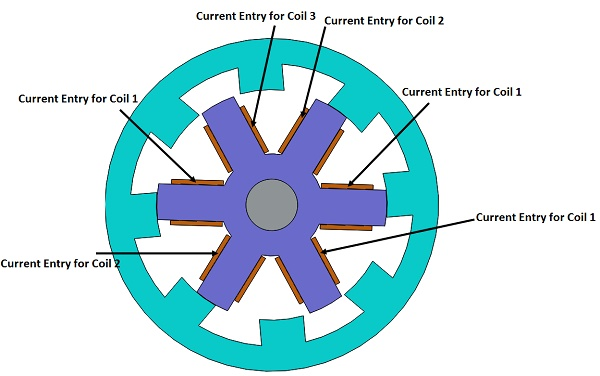Figure 2 - Current entry ports for the three wound coils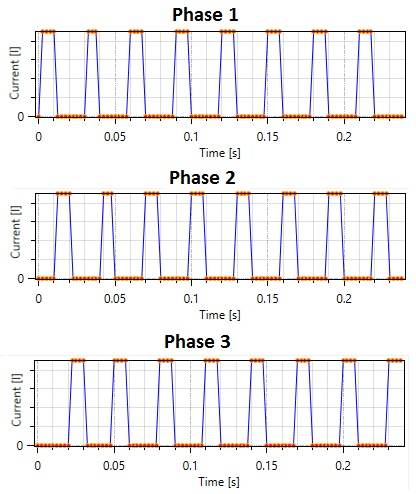Figure 3 - Current excitation of the three phases

## Material

The simulated model composed of a stator, a rotor, a shaft, coils, an Inner Air, a Band and an Outer Air. The properties of the materials are summarized in Table 1.

Table 1: Materials used in the EMS simulation

 Component Material Relative permeability Conductivity (S/m) Stator, rotor, shaft M-19 Non linear 0 Coils Copper 0.99991 5.8e+007 Band, Inner Air, Outer Air Air 1 0

## Magnetic flux density computation

In the design of a switched reluctance motor, magnetic flux density is a crucial parameter in the choice of the suitable ferromagnetic cores material. EMS computes the magnetic flux density for each time step (rotor position).
As compared to reference , the magnetic flux density maximum value was found to be 2.11 Tesla as compared in Table 2.

Table 2: Magnetic flux density calculated by EMS and compared to Reference 

 Parameter EMS result Reference  result Magnetic flux density maximum value 2.11 Tesla 1.9 Tesla

## 3D fields generated by the Switched Reluctance Motor

EMS creates 3D plots of the magnetic flux density. Figure 4 and 5 show the 3D vector plot and the 3D plot of the magnetic flux density, respectively.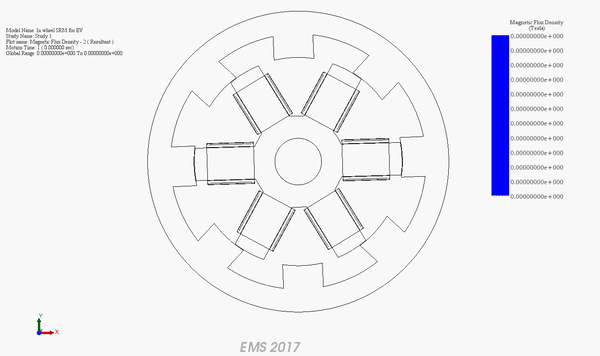Figure 4 - 3D Vector plot of magnetic flux density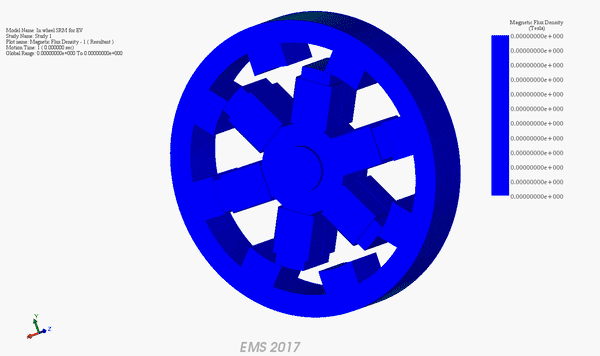Figure 5 - 3D plot of the magnetic flux density

## Conclusion

This EMS can be used in conjunction with SolidWorks Motion to study Electrical machines like Switched Reluctance Motor. Using EMS, and for each rotor position, magnetic flux density, magnetic field intensity etc can easily computed and visualized in 3D.

## References

 K. Cakir A. Sabanovic, “In-wheel Motor Design for Electric Vehicles” 9th IEEE International Workshop on Advanced Motion Control, 2006.